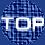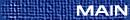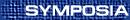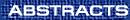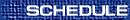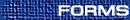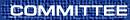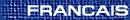Rigorous Studies in the Statistical Mechanics of Lattice Models / Études rigoureuses dans la mécanique statistique des modèles de réseaux
(Chris Soteros and Stu Whittington, Organizers)

MIREILLE BOUSQUET-MÉLOU, LaBRI, Universite Bordeaux 1. 351 cours de la Liberation. 33405  Talence Cedex. France
The site-perimeter of bargraphs

The site perimeter of a lattice animal is the number of vertices of the underlying grid that are adjacent to the animal (but do not belong to it). This parameter plays an important role in percolation models.

As self-avoiding polygons can also be seen as polyominoes (hence animals), one can also study their site perimeter. The case of polygons that are both column- and row-convex is easy to deal with, and we shall explain why it always yields an algebraic generating function. But the main result of the talk will be the exact solution of a model of polygons that are ONLY column-convex: bargraphs. This model can also be described as a self-interacting partially directed walk attached to a surface.

We obtain the site perimeter generating function for bargraphs as a q-series in which an algebraic series is plugged: to our knowledge, this type of series has not appeared before in the zoo of animals generating functions.

(joint work with Andrew Rechnitzer)

RICHARD BRAK, Melbourne
Combinatorial aspects of the simple asymmetric exclusion process

The simple asymmetric exclusion process (ASEP) is a finite one dimensional system of particles hopping with excluded volume. It is a dynamical model for problems such as driven diffusive systems, traffics flows and the kinetics of biopolymerization. Much work has been done on exact calculations for the stationary distribution for the many variants of the model. All these methods are all algebraic. In this talk we show that there is a very rich combinatorial dimension to the ASEP. The infinite dimensional matrices that arise as representations of the algebras in the problem can be interpreted as lattice path problems. This interpretation leads to combinatorial solutions to many of the problems. These solutions use new involutions and bijections. Interestingly, one of the lattice path representations is related to compact percolation with a damp wall.

FRANK DEN HOLLANDER, Eurandom, Eindhoven, The Netherlands
Heteropolymers near interfaces

We consider a heteropolymer, consisting of an i.i.d. concatenation of hydrophilic and hydrophobic monomers, in the presence of water and oil arranged in alternating layers. The heteropolymer is modelled by a directed path

 (i,Si)i Î N0,
where the vertical component lives on Z, and the layers are horizontal with equal width. The path measure for the vertical component is given by that of simple random walk multiplied by an exponential weight factor that favors the combinations hydrophilic/water and hydrophobic/oil and disfavors the combinations hydrophilic/oil and hydrophobic/water. We study the vertical motion of the heteropolymer as a function of its total length n when the width of the layers is dn and the parameters in the exponential weight factor are such that the heteropolymer tends to stay close to an interface (the so-called ``localized regime''). In the limit as n ®¥ and under the condition that

 lim n ® ¥ dnloglogn = ¥, lim n ® ¥ dnlogn = 0,
we show that the vertical motion is a diffusive hopping between neighboring interfaces on a time scale

 exp[cdn+o(dn)],
where c is computed explicitly in terms of a variational problem. An analysis of this variational problem sheds light on the optimal hopping strategy.

(Joint work with M. Wüthrich, Winterthur, Switzerland.)

YUANAN DIAO, University of North Carolina at Charlotte, Charlotte, North Carolina  28269, USA

The maximum of the linking number between two lattice polygons of lengths n1, n2 (with n1 £ n2) is proven to be of the order of n1(n2)[1/3]. This result is generalized to smooth links of unit thickness. The result also implies that the writhe of a lattice knot K of length n is at most 26n4/3 /p. In the second half of the paper examples are given to show that linking numbers of order n1(n2)[1/3] can be obtained when n13 ³ n2. When n13 < n2, it is further shown that the maximum of the linking number between these two polygons is bounded by cn12 for some constant c > 0. Finally the maximal total linking number of lattice links with more than 2 components is generalized to k components.

BERTRAND DUPLANTIER, Institut Henri Poincaré 11, rue Pierre et Marie Curie, F-75231 Paris Cedex 05 and Service de Physique Théorique, CEA/ Saclay, F-91191  Gif-Sur-Yvette Cedex, France
Distribution of potential near conformally invariant boundaries

The distribution of the electrostatic potential near any conformally invariant fractal boundary is exactly solved in two dimensions. This class of boundaries appears in a natural way in the statistical mechanics of any random cluster at a critical point, like a percolation or an Ising cluster, a Brownian path, or a self-avoiding walk.

Consider a single charged random cluster, generically called C. Let H (z) be the potential at exterior point z, with Dirichlet boundary conditions H(w Î C)=0 on the outer boundary C of C. The multifractal formalism characterizes subsets Ca of boundary sites w by a local exponent a, such that the potential scales at distance r=|z-w| from w as

 H (r| w Î ¶Ca) » r a,     r ® 0.
(1)
The subset Ca has a varying Hausdorff ``multifractal'' dimension f(a)=dim(Ca). An electrostatic exponent a corresponds geometrically to a local equivalent opening angle q = p/a along the fractal boundary, and the Hausdorff dimension [^(f)](q)=f(a = p/q) of the boundary subset with such angle q is found to be

 ^f (q)= pq - 25-c12 (p-q)2q(2p-q) ,
(2)
with c is a parameter describing the critical model. This naturally solves the potential theory near a random fractal in a statistical, i.e., probabilistic sense. Surprisingly, this classical problem is solved in the simplest way by a method of ``quantum gravity'', borrowed from string theory.

Values of c are, for instance, c=1/2 for an Ising cluster; c=0 for the frontier of a Brownian motion , for a self-avoiding walk , as well as for a critical percolation cluster . One thus finds for c=0 that these three boundaries all have the statistics of a self-avoiding walk, with a unique external perimeter dimension DEP=supq[^(f)](q)=4/3, which establishes and generalizes a well-known conjecture by Mandelbrot. This dimension is identical to the external perimeter dimension for percolation, directly derived in .

For any value of c, the Hausdorff dimension of the frontier DEP = supq[^(f)](q)=[^(f)]([^(q)]), and the typical harmonic angle [^(q)] satisfy [^(q)]=p(3-2DEP). For a critical Potts cluster, the dimensions DEP of the external perimeter (which is a simple curve) and DH of the cluster's hull (which possesses double points) obey the duality equation 

 (DEP-1)(DH-1)= 14 ,
(3)
independently of the model.

A related covariant MF spectrum is obtained for any critical system near the cluster boundary.

References

{1.} B. Duplantier, Conformally Invariant Fractals and Potential Theory. Phy. Rev. Lett. 84(2000), 1363-1367.
{2.}      , Random Walks and Quantum Gravity in Two Dimensions. Phy. Rev. Lett. 81(1998), 5489-5492.
{3.}      , Two-Dimensional Copolymers and Exact Conformal Multifractality. Phy. Rev. Lett. 82(1999), 880-883.
{4.}      , Harmonic Measure Exponents for 2D Percolation. Phy. Rev. Lett. 82(1999), 3940-3943.
{5.} A. Aizenman, B. Duplantier and A. Aharony, Path Crossing Exponents and the External Perimeter in 2D Percolation. Phys. Rev. Lett. 83(1999), 1359-1362.

TONY GUTTMANN, The University of Melbourne, Victoria  3010, Australia
The scaling function of self-avoiding polygons

We derive the scaling function for rooted self-avoiding polygons, on the square and triangular lattices, enumerated by both perimeter and area, defined up to a single constant. The scaling function satisfies a Ricatti equation, and its solution is given by the logarithmic derivative of an Airy function. The scaling function for unrooted self-avoiding polygons is given by the logarithm of an Airy function. While believed to be exact, the results are not rigorous. The result suggests that the generating function may be the solution of a q-algebraic difference equation of unknown degree. For two-dimensional percolation theory, by contrast, the generating function cannot be expressed as a q-algebraic difference equation.

Critical exponents for trails in Z2 with a fixed number of vertices of degree 4

For N large, the possible number of N-edge embeddings in Zd of an arbitrary connected graph t, is believed to grow asymptotically as At ekN Ngt-1, where At is a constant, depending on the type of graph t (a walk, polygon, figure-eight, etc.), and k and gt are called the connective constant and critical exponent, respectively. Assuming the above asymptotic form, we prove for d = 2, that the critical exponent for trailgons (closed trails) with k vertices of degree 4 is given by go + k, where go is the critical exponent for self-avoiding polygons. A similar relation holds between the critical exponents for all trails with k vertices of degree 4 and for self-avoiding walks.

BUKS JANSE VAN RENSBURG, York University, Toronto, Ontario  M3J 1P3

A model for a ring polymer adsorbing onto a solid wall is a polygon confined in the half space z ³ 0 of the cubic lattice, and adsorbing onto the z=0 plane. Such a polygon is also an embedding of the circle in real space, and its knot type is well defined. In this talk I shall present rigorous and numerical results (including the energy and mean size) of adsorbing polygons, with particular reference to the entanglement complexity of these polygons, as measured by considering their knot types. Numerical data were obtained by a multiple Markov Chain implementation of the pivot algorithm for polygons confined in half-space z ³ 0 interacting with the z=0 plane and in the canonical ensemble.

ANTAL JARAI, PIMS-UBC, 1933 West Mall, Vancouver, British Columbia  V6T 1Z2
Incipient infinite clusters in 2D percolation

Percolation models at the critical point produce huge but finite connected clusters that have sometimes been referred to as ``incipient infinite clusters'' or ``infinite clusters at criticality''. In 1986 H. Kesten proposed the definition of an object, which we call the IIC, that makes sense of the above loose terminology. In his definition the origin is conditioned to be connected to the boundary of a large box (at p = pc), and the size of the box goes to infinity. In 2D the conditional measures can be shown to have a weak limit, and under the limiting measure the cluster of the origin is an infinite fractal-like set resembling the large critical clusters.

As noted by M. Aizenman, the IIC provides the microscopic (lattice scale) description of large percolation clusters at pc. In this talk we give a proof of this observation in several settings. For example, consider the largest cluster in a finite box and pick a site from it uniformly at random. Then we show that the law of the cluster, when viewed from the random site, converges weakly to the IIC as the box gets large. Similar results hold, if the largest cluster is replaced by spanning clusters, the Chayes-Chayes-Durrett cluster or the invaded region in invasion percolation. All of these objects have been proposed as alternative definitions of the ``incipient infinite cluster''; therefore our results show the equivalence of several natural definitions of the IIC.

PIERRE LEROUX, Université du Québec, Montréal, Québec
Enumeration of symmetry classes of convex and parallelogram polyominoes

Several families of polynominoes, defined by convexity or directedness properties, have been enumerated according to area, perimeter and more refined parameters, on the square lattice. In this context, polyominoes are taken up to translations. However, it is also natural, from a geometric point of view, to consider polyominoes up to rotations and reflections, that is as ``tiles'' which can move freely in space. These congruence-type polyominoes can be seen as orbits of the dihedral group D4 acting on (translation-type) polyominoes. Using Burnside's Lemma, we are led into the enumeration of the various symmetry classes of polyominoes associated with each element of D4. Together with my students E. Rassart and A. Robitaille, we have solved this problem in the cases of convex and of parallelogram polyominoes. Using Moebius inversion, we have also enumerated the asymmetric polyominoes of these two sorts. Many of the symmetry classes which occur are closely related to existing families of discrete models in statistical mechanics, for example compact source directed convex polyominoes, and their study raises challenging questions. In this talk, I will survey this work and mention some open problems.

NEAL MADRAS, Department of Mathematics and Statistics, York University, Toronto, Ontario  M3J 1P3
Self-avoiding walks on hyperbolic graphs

This talk considers graphs that correspond to regular tilings of the hyperbolic plane. One example is the infinite planar graph in which every face is a triangle and eight triangles meet at every vertex. To a physicist, such graphs display ``infinite dimensional'' characteristics. In particular, self-avoiding walks should behave like ordinary random walks on these graphs. This predicts, for example, that there is exponentially small probability for a random N-step self-avoiding walk to end at a neighbour of the initial point. I shall discuss work in this direction.

ALEKS OWCZAREK, The University of Melbourne, Victoria, Australia  3010
An infinite hierarchy of exact scaling functions

The partition functions for various problems concerning n directed non-intersecting walks on a square lattice are known to be given by an n×n determinant. The analysis of the asymptotic behaviour is often an outstanding problem because of the difficulty with analysing such an expression. In this talk we focus on the particular problem of such walks interacting via contact potentials with a wall parallel to the direction of the walks, and derive the asymptotics of the partition function of a watermelon network of n such walks for all interaction parameters. The importance of underlying combinatorial results are highlighted in this calculation. As a result we give results for the associated network exponents in the three regimes: desorbed, adsorbed, and at the adsorption transition. Furthermore, we derive the full scaling function around the adsorption transition for all n. At the adsorption transition we also derive a simple ``product form'' for the partition function.

NICHOLAS PIPPENGER, Computer Science Department, University of British Columbia, Vancouver, British Columbia  V6T 1Z4
Random Boolean functions

We consider random Boolean functions of n arguments, f :Bn ® B (where B = {0, 1}), for which each value f(x1, ¼, xn) is independently 1 with probability p (and thus 0 with probability 1-p). We study the expected length [`(L)](n) of the shortest representation of such a function in disjunctive normal form (that is, as the disjunction of zero or more terms, each of which is the conjunction of zero or more literals, each of which is either an argument or its complement). The bounds we obtain for this problem involve correlation inequalities, cluster expansions, and other tools of statistical physics.

ANDREW RECHNITZER, Department of Mathematics and Statistics, York University, York, Ontario

One of the many places that lattice animals and their specialisations appear is in the modeling of the polymers in solution. The self-avoiding walk, for example, is used to model long chain polymers in solution; by altering the properties of the walk, different physical situations can be modeled, such as polymer collapse and adsorption.

Though the self-avoiding walk model is a very good model of such behaviour, it has one major draw-back-it is (as yet) unsolved. It is possible to obtain a solvable model by considering directed-walk models, such as Dyck-paths.

We review the methods for solving the Dyck-path model, and show that it undergoes an adsorption transition. We then extend this model to the problem of non-homogeneous polymers and explain the difficulty of finding general solutions. For an infinite family of cases we are able to find solutions and locate the adsorption transition.

YVAN SAINT-AUBIN, Université de Montréal, CP 6128 succ centre-ville, Montréal, Quebéc  H3C 3J7
Non-unitarity of some observables of the critical 2d Ising model

The distribution of spins at the end of a half-infinite cylinder is obtained rigorously for the critical 2d Ising model on a square lattice. As the number of sites along the circumference goes to infinity, the ratio of number of sign flips to number of sites at the boundary tends to a constant. Using conformal field theory, one can ask the finer question, which pair of sign flips at the boundary of the cylinder are the endpoints of the same cluster inside the cylinder. This leads to a critical exponent related to a non-unitary representation of the Virasoro algebra.

GORDON SLADE, Department of Mathematics, University of British Columbia Vancouver, British Columbia  V6T 1Z2
High-dimensional networks of self-avoiding walks

We discuss recent work with Remco van der Hofstad that proves that a sufficiently spread-out network of mutually-avoiding self-avoiding walks having the structure of a tree behaves like a system of independent Brownian motions above four dimensions. Extensions to networks having the shape of a general graph, rather than a tree, will also be discussed. The proof uses a generalisation of the lace expansion, in which the time variable is indexed by a tree or a graph rather than by an interval.

ALAN SOKAL, New York University, New York, New York, USA
Potts models, chromatic polynomials, and all that

The q-state Potts model is a statistical-mechanical model that generalizes the well-known Ising model. It can be defined on an arbitrary finite graph, and its partition function encodes much important information about that graph (including its chromatic polynomial and its reliability polynomial). The complex zeros of the Potts-model partition function are of interest both to statistical mechanicians (in connection with the Lee-Yang picture of phase transitions) and to combinatorists. I begin by giving an introduction to all these problems. I then sketch two recent results: (a)  Proof of a universal upper bound on the q-plane zeros of the chromatic polynomial (or antiferromagnetic Potts-model partition function) in terms of the graph's maximum degree (maximum number of nearest neighbors to any site). (b)  Construction of a countable family of planar graphs whose chromatic zeros are dense in the whole complex q-plane except possibly for the disc |q-1| < 1. This talk is intended to be understandable to both mathematicians and physicists; no prior knowledge of either graph theory or statistical mechanics is required.

Random knotting and linking in lattice models of polymers

Self-avoiding polygons in Z3 can be used to represent the possible conformations of a ring polymer. Studies of random knotting in such a lattice model have been motivated by the conjecture (due to Frisch and Wasserman (1968) and Delbruck (1962)) that almost all sufficiently long ring polymers are knotted, and more recently by evidence that the presence of knots in closed circular DNA can provide information about enzyme action on DNA. More generally, embeddings of graphs (not necessarily connected) in Z3 can be used to represent the possible conformations of a polymer network, however, it remains a challenge to appropriately characterize the entanglement complexity of such networks. This has motivated studies of random linking in systems of self-avoiding polygons and random knotting in embeddings of graphs.

Recent rigorous results about random knotting and linking in lattice models of polymers will be reviewed. The results are focussed on understanding i)  the effects of geometrical constraints on the probability of knotting in self-avoiding polygons, ii)  the probability of linking in systems of k self-avoiding polygons, and iii)  the extent to which these results can be extended to the study of polymer networks.

DE WITT SUMNERS, Florida State University, Tallahassee, Florida, USA
Using knots to analyze DNA packing in viral capsids

Bacteriophages are viruses that infect bacteria; the linear phage DNA is tightly packed in a protein icosahedron. When the viral DNA is released from the confining capsid, it circularizes, and these DNA circles are knotted with very high (80-95%) probability. We use the spectrum of DNA knots produced, together with simulation of knots in confined volumes to infer packing geometry. In particular, we conclude that DNA packing in the viral capsid is not random.

STU WHITTINGTON, Department of Chemistry, University of Toronto, Toronto, Ontario
Localization transition in a randomly coloured self-avoiding walk

Consider a self-avoiding walk on the simple cubic lattice with vertices coloured A and B uniformly and independently. The walk is assigned a weight which is determined by the number of A vertices having positive z-coordinate and the number of B vertices having negative z-coordinate. This can be regarded as a model of a random copolymer with two types of monomers, each of which prefers one of two immiscible solvents. We shall discuss the existence of the limiting quenched average free energy for this model, and thermodynamic self-averaging. The system can be shown to undergo a phase transition corresponding to localization at the interface (i.e. to a phase in which a non-zero fraction of the vertices of the walk have zero z-coordinate). Recent results about the nature of the phase diagram will be presented.